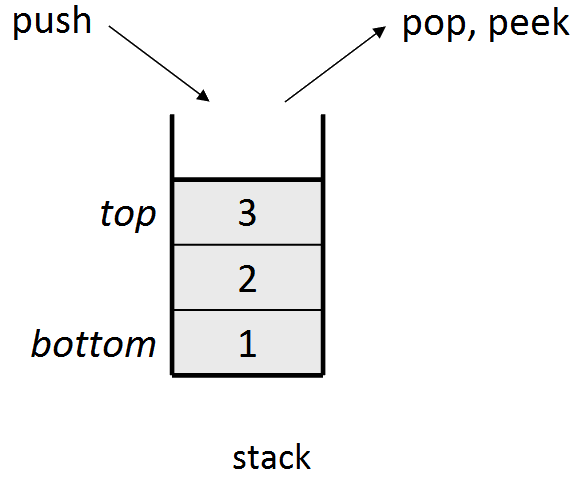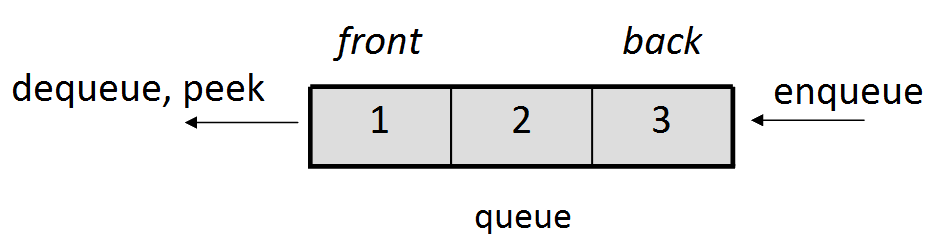### Lecture Preview: Stacks and Queues

(Suggested book reading: Programming Abstractions in C++, section 5.2 - 5.3)

In this lecture we will talk about two new data structures:

• A stack is a collection where you add elements to the "top" of the ordering, and remove from the "top" as well. The only accessible element is the one added latest. A stack is sometimes called a Last In, First Out ("LIFO") structure.• A queue is a collection where you add elements to the "back" of the ordering, and remove from the "front" of the ordering. The only accessible element is the one added earliest. A queue is sometimes called a First In, First Out ("FIFO") structure.When you declare a stack or queue, you write the type of data it will contain in `< >` brackets. Here is a short code example that uses a stack:

```Stack<int> s;                  // {}     bottom -> top
s.push(42);                    // {42}
s.push(-3);                    // {42, -3}
s.push(17);                    // {42, -3, 17}

cout << s.pop() << endl;       // 17   (s is {42, -3})
cout << s.peek() << endl;      // -3   (s is {42, -3})
cout << s.pop() << endl;       // -3   (s is {42})
```

Here is a short code example that uses a queue:

```Queue<int> q;                  // {}   front -> back
q.enqueue(42);                 // {42}
q.enqueue(-3);                 // {42, -3}
q.enqueue(17);                 // {42, -3, 17}
cout << q.dequeue() << endl;   // 42   (q is {-3, 17})
cout << q.peek() << endl;      // -3   (q is {-3, 17})
cout << q.dequeue() << endl;   // -3   (q is {17})
```
This document and its content are copyright © Marty Stepp, 2017. All rights reserved. Any redistribution, reproduction, transmission, or storage of part or all of the contents in any form is prohibited without the authors' expressed written permission.